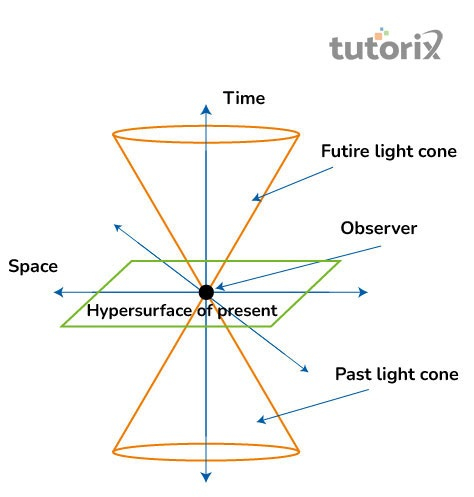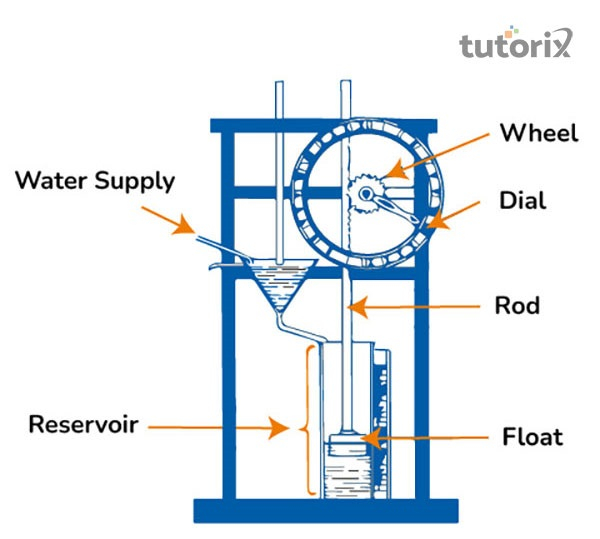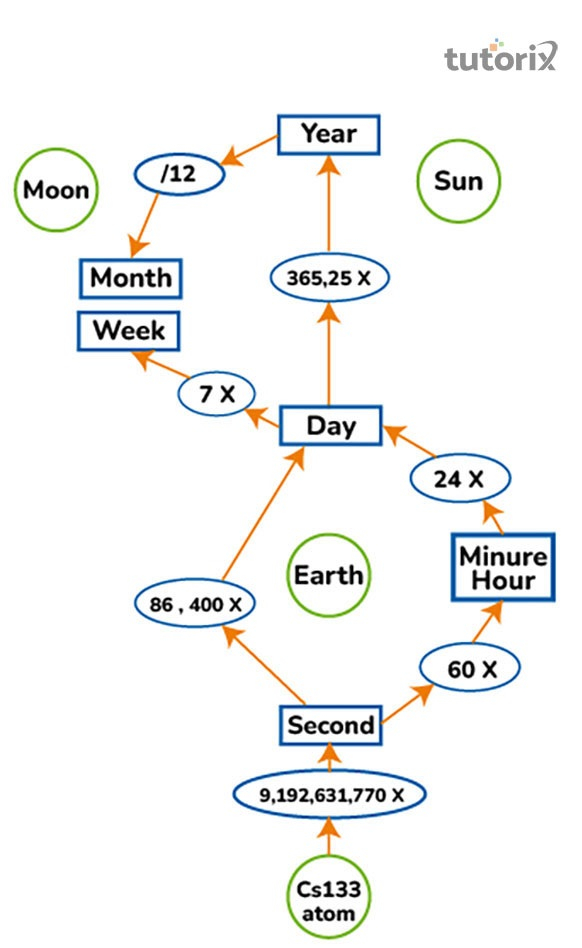# Time Measurement

## Introduction

It is quite difficult to define the aspect of time, but it is referred to as the measurable quantity that displays a series of ongoing and continuous sequences for events. It is noted that measuring time was not something new and from ancient times, several inventions were conducted.

As per the records, in ancient Egypt, inventions of sundials were to be found that was before''1500 .

It needs to be noted that the measurement of time calculated now is quite different from those times in ancient Egypt, with their different parameters.

## Definition of timeFigure 1: Theory of special relativity

In defining time, it is stated that time is not constant and it suffers continuous changes. Time can be referred to as a measurable parameter, always in a continuous motion. Time can be determined as the presence of both forces and motions that are majorly caused due to the successive expansion of space (Anwer et al. 2021).

However, these forces consist of both the kinetic and potential energies. Time is always a spatial continuum that is generally measured based on the events that change from one to another. In simpler words, events succeed from the past events to the present.

## Units of time

Several units of time are noticed all throughout the ages measuring the notion of time that includes, hour, seconds and minutes. On a broader aspect, other units that constitute, days, weeks, months and years respectively (Assef et al. 2018), can also denote time.

However, other measurements are there, such as past, present and lastly, future. All these are measured based on the events that are to be measured based on a given point of time on a reference frame.

According to the (SI) or International System of Units, the unit of time is expressed as, in the unit of the second.

In addition, the relationship that is noted for seconds, hours, and minutes is expressed as follows. 60 seconds is equal to 1 minute that in turn, 60 minutes can be expressed as, 1 hour. Therefore, 24 hours states a day (Smartick, 2022). These expressions can be determined as the conversations that are helpful for mathematical expressions.

## Relationship between time and periodic motion

There lies an intricate relationship between the notions of time and periodic motions. Periodic motion is related to the motion of respective objects that intend to repeat themselves, after a specific period of time. Now the total number of repetitions for several movements based on the every second is termed frequency. (Exactlywhattime, 2022). Therefore, both the aspects of time and periodic are expressions as, (f) = 1/T, where, f denotes frequency, and T represents time period.

## Ancient devices for time measurement

Several time measuring instruments are invented over time and some of the popular ones include, sundials, water clocks or candle clocks, sandglass, pendulum and quartz. Einstein developed Quartz during the 1930s and Galileo Galili developed the concept of pendulum.

The usage of sundials was, however based on the time intervals between sunrise and sunsets.Figure 2: Water Clocks

## Formula and mathematical representation of time

In the field of physics, the notion of time can be determined as the measurements determined by distance and speed respectively.

However, in simpler words, it is defined as the distance travelled by an object with a particular speed. However, the above statements can be expressed as, time = distance/speed for a particular object. Time measurements are done with several aspects based on the conversion table.Figure 3: Interrelationships among the major units of time

## Examples of time measurements

Some examples are as follows that will support in providing a method for measuring time. For example, how many minutes are there in 1000 seconds, therefore, the result will be 1000/60 and that equals 16 minutes and 40 seconds.

Another such example is, how many minutes are there within three hours?, therefore, 3*60 that results in 180 minutes. Then again, what is the total time covered by 3600m at 2 meters per seconds?, therefore, as per the formula, Time = Distance ÷ Speed results in 3600/2 and the final answer is 1800 seconds.

## Conclusion

In this tutorial, the concept of time is being discussed catering to the associative measurements that are used as units. However, it is noticed that there lie many units for the notion of time. They have an intricate relationship and seconds being the unit of time determines an expression with other aspects that are associated with time.

Q1. What is known as time dilation?

Ans: Einstein can express Time dilation through the theory of relativity. The term time dilation refers to the differences that are noticed between the two respective events and their elapses in time. This elapsed time is measured through the relative movement towards each other and that are located variedly from the masses of gravitation. Several devices of time are invented from the ancient ages and are stated to be invented in ancient Egypt.

Q2. What is the theory of special relativity?

Ans: Theory of special relativity is majorly a theory of gravity and it defines gravity as the curving and wrapping of space. This furthermore proves that gravity is not an invisible force that tends to attract one object towards another object.

Q3. What is defined as clepsydra?

Ans: In ancient times, before the invention of the modern system of clocks, water clocks were invented. These water clocks are used to be known as clepsydra and these were invented in 1500 BCE ''. However, these time clocks used to work on maintaining the steady flow of water from one container to another container. Afterwards, the measurements are marked on the counters that are used and this is how the time was measured. However, this device used to be more reliable than the candle or other lamps of oil.